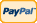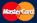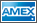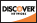•Electronics, electrical engineering
•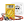Engineering physics
•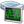Generators
•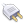Interfaces
•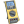Multimeters
•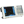Oscilloscopes
•Persona
•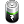Power supplies
•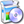Software
•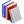Standards
•Thesaurus
•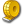Units of measurement
Filter by first letter
A
B
C
D
E
F
G
H
I
J
K
L
M
N
O
P
Q
R
S
T
U
V
W
X
Y
Z
+
-

# Second (s)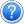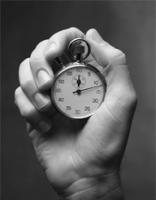The second (SI symbol: s), sometimes abbreviated sec., is a unit of measurement of time, and is the International System of Units (SI) base unit of time. It may be measured using a clock.

Early definitions of the second were based on the apparent motion of the sun around the earth. The solar day was divided into 24 hours, each of which contained 60 minutes of 60 seconds each, so the second was 186400 of the mean solar day. However, 19th- and 20th century astronomical observations revealed that this average time is lengthening, and thus the sun/earth motion is no longer considered a suitable basis for definition. With the advent of atomic clocks, it became feasible to define the second based on fundamental properties of nature. Since 1967, the second has been defined to be the duration of 9,192,631,770 periods of the radiation corresponding to the transition between the two hyperfine levels of the ground state of the caesium 133 atom.

SI prefixes are frequently combined with the word second to denote subdivisions of the second, e.g., the millisecond (one thousandth of a second), the microsecond (one millionth of a second), and the nanosecond (one billionth of a second). Though SI prefixes may also be used to form multiples of the second such as kilosecond (one thousand seconds), such units are rarely used in practice. The more common larger non-SI units of time are not formed by powers of ten; instead, the second is multiplied by 60 to form a minute, which is multiplied by 60 to form an hour, which is multiplied by 24 to form a day.

The second is also the base unit of time in the centimetre-gram-second, metre-kilogram-second, metre-tonne-second, and foot-pound-second systems of units.

Find more here: http://en.wikipedia.org/wiki/Second

Back to the list

Units Converter
• How it Works
• Company News
• Industry News & Events
• Measurement Fun FactsSite map|Privacy policy|Terms of Use & Store Policies|How to Buy|Shipping|Payment|© T&M Atlantic, Inc., 2010-2022